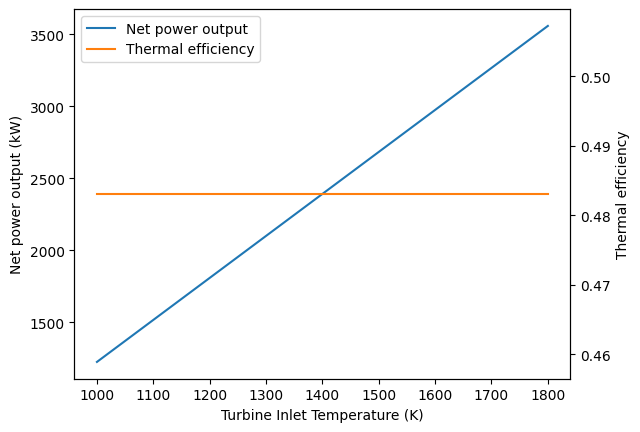# Cold-Air Standard Brayton Cycle Example¶

## Imports¶

:

from thermostate import State, Q_, units
import numpy as np

%matplotlib inline
import matplotlib.pyplot as plt


## Definitions¶

:

substance = "air"
p_1 = Q_(1.0, "bar")
T_1 = Q_(300.0, "K")
mdot = Q_(6.0, "kg/s")
T_3 = Q_(1400.0, "K")
p2_p1 = Q_(10.0, "dimensionless")
T_3_low = Q_(1000.0, "K")
T_3_high = Q_(1800.0, "K")


## Problem Statement¶

An ideal cold air-standard Brayton cycle operates at steady state with compressor inlet conditions of 300.0 kelvin and 1.0 bar and a fixed turbine inlet temperature of 1400.0 kelvin and a compressor pressure ratio of 10.0 dimensionless. The mass flow rate of the air is 6.0 kilogram / second. For the cycle,

1. determine the back work ratio

2. determine the net power output, in kW

3. determine the thermal efficiency

4. plot the net power output, in kW, and the thermal efficiency, as a function of the turbine inlet temperature from 1000.0 kelvin to 1800.0 kelvin. Discuss any trends you find.

## Solution¶

### 1. the back work ratio¶

In the ideal Brayton cycle, work occurs in the isentropic compression and expansion. Therefore, the works are

\begin{aligned} \frac{\dot{W}_c}{\dot{m}} &= h_1 - h_2 = c_p(T_1 - T_2) & \frac{\dot{W}_t}{\dot{m}} &= h_3 - h_4 = c_p(T_3 - T_4) \end{aligned}

First, fixing the four states using a cold air-standard analysis

:

st_amb = State(substance, T=T_1, p=p_1)
c_v = st_amb.cv
c_p = st_amb.cp
k = c_p / c_v

T_2 = T_1 * p2_p1 ** ((k - 1) / k)
p_2 = p2_p1 * p_1

p_3 = p_2

p_4 = p_1
T_4 = T_3 * (p_4 / p_3) ** ((k - 1) / k)


Summarizing the states,

State

T

p

1

300.00 K

1.00 bar

2

580.34 K

10.00 bar

3

1400.00 K

10.00 bar

4

723.71 K

1.00 bar

Then, the back work ratio can be found by

:

Wdot_c = (mdot * c_p * (T_1 - T_2)).to("kW")
Wdot_t = (mdot * c_p * (T_3 - T_4)).to("kW")
bwr = abs(Wdot_c) / Wdot_t


Answer: The power outputs are $$\dot{W}_c =$$ -1692.75 kW, $$\dot{W}_t =$$ 4083.53 kW, and the back work ratio is $$\mathrm{bwr} =$$ 0.41 = 41.45%

### 2. the net power output¶

:

Wdot_net = Wdot_c + Wdot_t


Answer: The net power output is $$\dot{W}_{net} =$$ 2390.78 kW

### 3. the thermal efficiency¶

:

Qdot_23 = (mdot * c_p * (T_3 - T_2)).to("kW")
eta = Wdot_net / Qdot_23


Answer: The thermal efficiency is $$\eta =$$ 0.48 = 48.31%

### 4. plot the net power output and thermal efficiency¶

:

T_range = np.linspace(T_3_low, T_3_high, 200)
Wdot_net_l = np.zeros(T_range.shape) * units.kW
eta_l = np.zeros(T_range.shape) * units.dimensionless
for i, T_3 in enumerate(T_range):
T_4 = T_3 * (p_4 / p_3) ** ((k - 1) / k)
Wdot_t = (mdot * c_p * (T_3 - T_4)).to("kW")
Wdot_net = Wdot_c + Wdot_t
Wdot_net_l[i] = Wdot_net

Qdot_23 = (mdot * c_p * (T_3 - T_2)).to("kW")
eta = Wdot_net / Qdot_23
eta_l[i] = eta

:

fig, power_ax = plt.subplots()
power_ax.plot(T_range, Wdot_net_l, label="Net power output", color="C0")
eta_ax = power_ax.twinx()
eta_ax.plot(T_range, eta_l, label="Thermal efficiency", color="C1")
power_ax.set_xlabel("Turbine Inlet Temperature (K)")
power_ax.set_ylabel("Net power output (kW)")
eta_ax.set_ylabel("Thermal efficiency")
lines, labels = power_ax.get_legend_handles_labels()
lines2, labels2 = eta_ax.get_legend_handles_labels()
power_ax.legend(lines + lines2, labels + labels2, loc="best");From this graph, we note that for a fixed compressor pressure ratio, the thermal efficiency is constant, while the net power output increases with increasing turbine temperature.